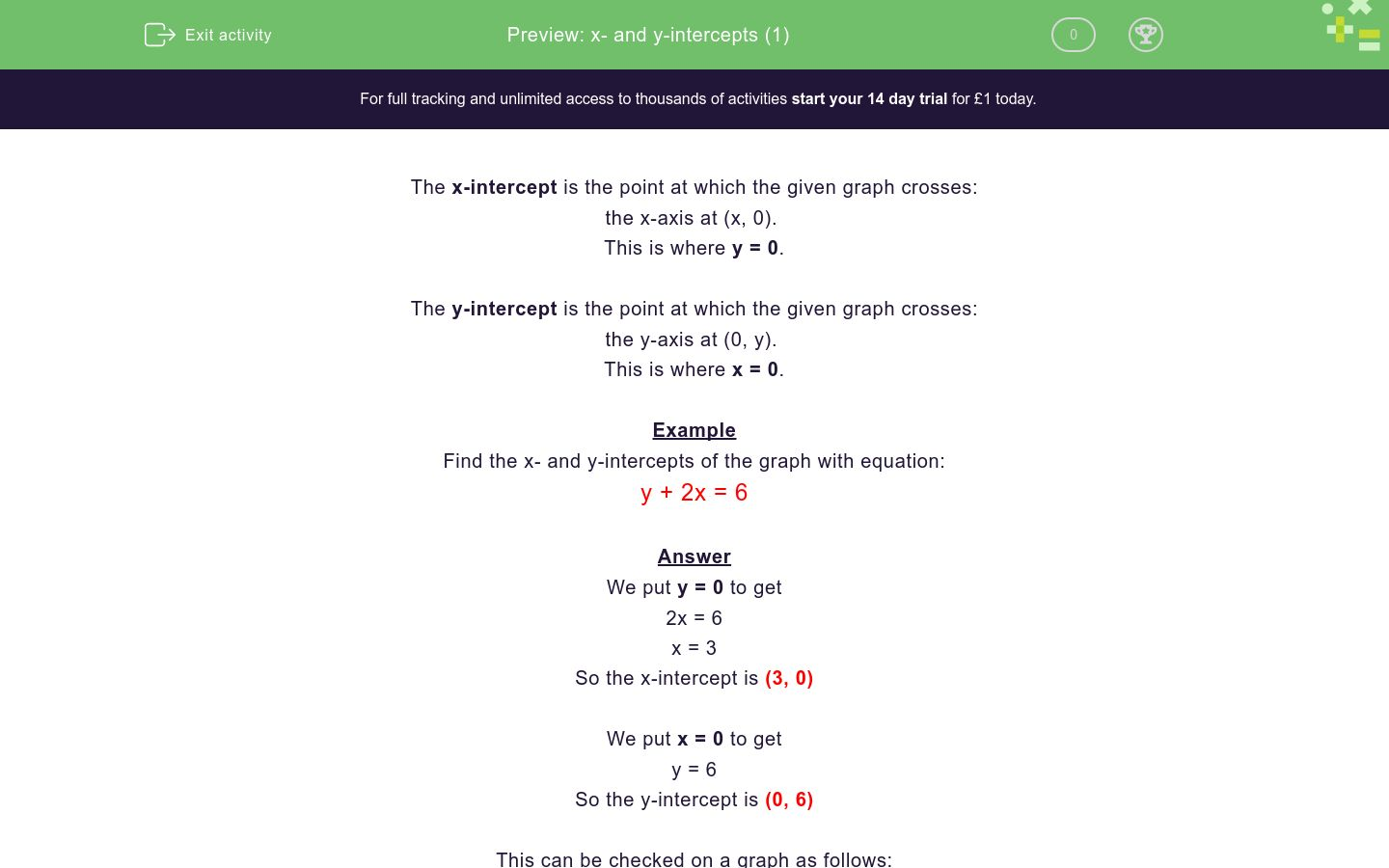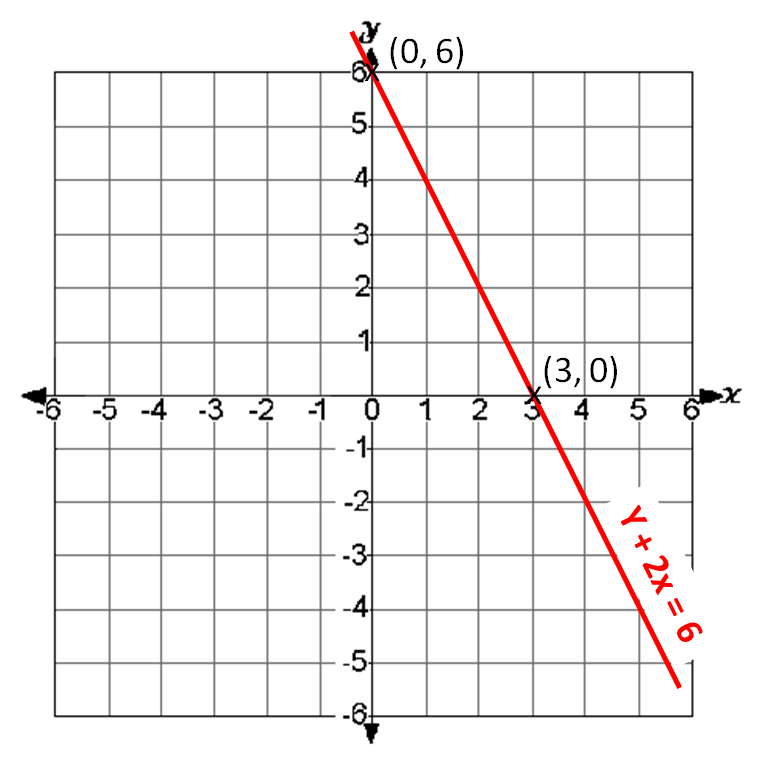# x- and y-intercepts (1)

In this worksheet, students must find the x- or y-intercepts of the given linear graph equations.Key stage:  KS 4

Curriculum topic:  Algebra

Difficulty level:### QUESTION 1 of 10

The x-intercept is the point at which the given graph crosses:

the x-axis at (x, 0).

This is where y = 0.

The y-intercept is the point at which the given graph crosses:

the y-axis at (0, y).

This is where x = 0.

Example

Find the x- and y-intercepts of the graph with equation:

y + 2x = 6

We put y = 0 to get

2x = 6

x = 3

So the x-intercept is (3, 0)

We put x = 0 to get

y = 6

So the y-intercept is (0, 6)

This can be checked on a graph as follows:Find the x-intercept of the graph with equation

y + 3x = 6

(0, 6)

(6, 0)

(2, 0)

(0, 2)

Find the y-intercept of the graph with equation

y + 3x = 6

(0, 6)

(6, 0)

(2, 0)

(0, 2)

Find the x-intercept of the graph with equation

y - 3x = 9

(0, 9)

(9, 0)

(3, 0)

(-3, 0)

Find the y-intercept of the graph with equation

y - 3x = 9

(9, 0)

(3, 0)

(-3, 0)

(0, 9)

Find the x-intercept of the graph with equation

2y + 3x = 6

(0, 6)

(6, 0)

(2, 0)

(0, 2)

Find the y-intercept of the graph with equation

2y + 3x = 6

(0, 3)

(6, 0)

(3, 0)

(0, 2)

Find the x-intercept of the graph with equation

2y - 3x = 12

(0, -4)

(-4, 0)

(4, 0)

(0, 4)

Find the y-intercept of the graph with equation

2y - 3x = 12

(0, 6)

(6, 0)

(2, 0)

(0, 2)

Find the x-intercept of the graph with equation

3x - 12y = 6

(0, 6)

(6, 0)

(2, 0)

(0, 2)

Find the y-intercept of the graph with equation

3x - 12y = 6

(0, -2)

(2, 0)

(-½, 0)

(0, -½)

• Question 1

Find the x-intercept of the graph with equation

y + 3x = 6

(2, 0)
EDDIE SAYS
When y = 0,
3x = 6
x = 2
• Question 2

Find the y-intercept of the graph with equation

y + 3x = 6

(0, 6)
EDDIE SAYS
When x = 0,
y = 6
• Question 3

Find the x-intercept of the graph with equation

y - 3x = 9

(-3, 0)
EDDIE SAYS
When y = 0,
-3x = 9
x = -3
• Question 4

Find the y-intercept of the graph with equation

y - 3x = 9

(0, 9)
EDDIE SAYS
When x = 0,
y = 9
• Question 5

Find the x-intercept of the graph with equation

2y + 3x = 6

(2, 0)
EDDIE SAYS
When y = 0,
3x = 6
x = 2
• Question 6

Find the y-intercept of the graph with equation

2y + 3x = 6

(0, 3)
EDDIE SAYS
When x = 0,
2y = 6
y = 3
• Question 7

Find the x-intercept of the graph with equation

2y - 3x = 12

(-4, 0)
EDDIE SAYS
When y = 0,
-3x = 12
x = -4
• Question 8

Find the y-intercept of the graph with equation

2y - 3x = 12

(0, 6)
EDDIE SAYS
When x = 0,
2y = 12
y = 6
• Question 9

Find the x-intercept of the graph with equation

3x - 12y = 6

(2, 0)
EDDIE SAYS
When y = 0,
3x = 6
x = 2
• Question 10

Find the y-intercept of the graph with equation

3x - 12y = 6

(0, -½)
EDDIE SAYS
When x = 0,
-12y = 6
y = -½
---- OR ----

Sign up for a £1 trial so you can track and measure your child's progress on this activity.

### What is EdPlace?

We're your National Curriculum aligned online education content provider helping each child succeed in English, maths and science from year 1 to GCSE. With an EdPlace account you’ll be able to track and measure progress, helping each child achieve their best. We build confidence and attainment by personalising each child’s learning at a level that suits them.